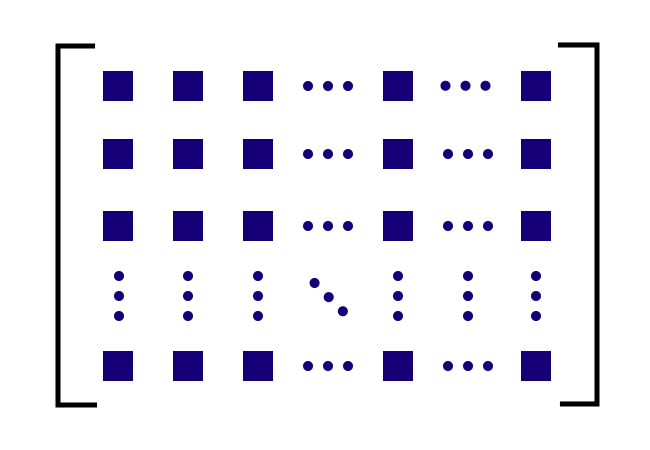# Main diagonal of a Rectangular matrix

A straight path that connects the entries (or elements) whose row and column are the same in a rectangular matrix is called the main diagonal of a rectangular matrix.

## Introduction

In a rectangular matrix, the total number of elements in a row is not equal to the total number of entries in a column but there is an element whose row and column are equal in every row or column of a rectangular matrix, those elements can be connected by a straight path diagonally and it is called a main diagonal of a rectangular matrix.The main diagonal of a rectangular matrix is also called by the following four names alternatively in mathematics.

1. Principal diagonal of a rectangular matrix
2. Primary diagonal of a rectangular matrix
3. Leading diagonal of a rectangular matrix
4. Major diagonal of a rectangular matrix

For example, we have some entries and they are arranged in $m$ rows and $n$ columns. The arrangement of the elements can be expressed in a matrix form as follows.

$M$ $\,=\,$ $\begin{bmatrix} \color{red} e_{11} & e_{12} & e_{13} & e_{14} & \cdots & e_{1m} \\ e_{21} & \color{red} e_{22} & e_{23} & e_{24} & \cdots & e_{2m} \\ e_{31} & e_{32} & \color{red} e_{33} & e_{34} & \cdots & e_{3m} \\ \vdots & \vdots & \vdots & \color{red} \ddots & \vdots & \vdots \\ e_{m1} & e_{m2} & e_{m3} & e_{m4} & \cdots & e_{mn} \\ \end{bmatrix}$

In this case, the total number of elements in a row is not equal to the total number of elements in a column. Hence, a rectangle shape is formed in the matrix and the matrix is called a rectangular matrix.

In the rectangular matrix $M$, the elements $e_{11}$, $e_{22}$, $e_{33}$, $e_{44}$ and so on can be connected diagonally by a straight path. Hence, the diagonal path is called the main diagonal of the rectangular matrix $M$.

### Examples

Let’s learn the concept of main diagonal of a rectangular matrix from two understandable examples.

$A$ $\,=\,$ $\begin{bmatrix} \color{red} 5 & 0 & 1 & 7 \\ 2 & \color{red} 3 & 9 & 8 \\ 3 & 5 & \color{red} 4 & 2 \\ \end{bmatrix}$

The matrix $A$ represents a rectangular matrix of the order $3 \times 4$. In this matrix, we have to identify the entries, whose row and column are the same. In the rectangular matrix $A$, the elements $e_{11} \,=\, 5$, $e_{22} \,=\, 3$ and $e_{33} \,=\, 4$.

Hence, the diagonal straight path that connects all of these elements is called the principal diagonal of the rectangular matrix $A$.

$B$ $\,=\,$ $\begin{bmatrix} \color{red} 4 & 0 & 2 & 1 & 7 \\ 6 & \color{red} 1 & 7 & 8 & 3 \\ 8 & 1 & \color{red} 7 & 9 & 2 \\ 3 & 5 & 7 & \color{red} 5 & 5 \\ 0 & 3 & 2 & 6 & \color{red} 6 \\ 7 & 4 & 3 & 6 & 3 \\ \end{bmatrix}$

The matrix $B$ is a rectangular matrix of the order $6 \times 5$. Now, identify the elements, whose row and column are the same. In the rectangular matrix $B$, $e_{11} \,=\, 4$, $e_{22} \,=\, 1$, $e_{33} \,=\, 7$, $e_{44} \,=\, 5$ and $e_{55} \,=\, 6$. Therefore, the straight path that connects these entries (or elements) diagonally is called the leading diagonal of the rectangular matrix $B$.

Latest Math Topics
Jun 26, 2023
Jun 23, 2023

Latest Math Problems
Jul 01, 2023
Jun 25, 2023
###### Math Questions

The math problems with solutions to learn how to solve a problem.

Learn solutions

Practice now

###### Math Videos

The math videos tutorials with visual graphics to learn every concept.

Watch now

###### Subscribe us

Get the latest math updates from the Math Doubts by subscribing us.